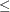### TYPE Statement

TYPE variable ;

CONTYPE variable ;

The TYPE list, which is optional, names the variable that has as values keywords that indicate either the constraint type for each constraint or the type of special rows in the CONDATA= data set. The values of the TYPE list variable also indicate, in each observation of the CONDATA= data set, how values of the VAR or COEF list variables are to be interpreted and how the type of each constraint or special row name is determined. If the TYPE list is not specified, the CONDATA= data set is searched and a SAS variable with the name _TYPE_ is used. Valid keywords for the TYPE variable are given below. If there is no TYPE statement and no other method is used to furnish type information (see the DEFCONTYPE= option), all constraints are assumed to be of the type less than or equal to and no special rows are used. The TYPE list variable must have character values and can be used when the data in the CONDATA= data set is in either the sparse or the dense format. If the TYPE list variable value has a * as its first character, the observation is ignored because it is a comment observation.

#### TYPE List Variable Values

The following are valid TYPE list variable values. The letters in boldface denote the characters that PROC NETFLOW uses to determine what type the value suggests. You need to have at least these characters. In the following list, the minimal TYPE list variable values have additional characters to aid you in remembering these values.

 < less than or equal to () = equal to (=) > greater than or equal to () CAPAC capacity COST cost EQ equal to FREE free row (used only for linear programs solved by interior point) GAIN gain in arc flow (generalized networks) GE greater than or equal to LE less than or equal to LOSS loss in arc flow (generalized networks) LOWERBD lower flow or value bound LOWblank lower flow or value bound MAXIMIZE maximize (opposite of cost) MINIMIZE minimize (same as cost) MULT value of arc multiplier (generalized networks) OBJECTIVE objective function (same as cost) RHS rhs of constraint TYPE type of constraint UPPCOST reserved for future use UNREST unrestricted variable (used only for linear programs solved by interior point) UPPER upper value bound or capacity; second letter must not be N

The valid TYPE list variable values in function order are

• LE less than or equal to ()

• EQ equal to (=)

• GE greater than or equal to ()

• COST MINIMIZE MAXIMIZE OBJECTIVE cost or objective function coefficient

• CAPAC UPPER capacity or upper value bound

• LOWERBD LOWblank lower flow or value bound

• RHS rhs of constraint

• TYPE type of constraint

• MULT GAIN LOSS value of arc multiplier in a generalized network

A TYPE list variable value that has the first charactercauses the observation to be treated as a comment. If the first character is a negative sign, thenis the type. If the first character is a zero, thenis the type. If the first character is a positive number, thenis the type.